Question

1. Why is Newton's Universal Law of Gravity still in use if we have a better theory such as Einstein's General Theory of Relativity?

2. What does each of Kepler's Laws say about planetary motion?

3. Describe why scientists (especially physicists and astronomers) believe we need a quantum theory of gravity. Where is this dilemma most problematic?

3. State each of Newton's three laws of motion and give an example of each. (You may find it simplest to google "Newton's Laws of Motion" and summarize each in your own words. Note that Newton's Universal Law of Gravity is an additional law, beyond the 3 laws of motion).

(1) Ans.

Why is Newton's Universal Law of Gravity still in use if we have a better theory such as Einstein's General Theory of Relativity?

Because newtons law of Gravitation was not proven wrong by einstien, but as incomplete for some factors….for daily life objects, these factors don't affect much thus can be seen and verified…but for much larger macroscopic objects, these factors can't be ignored as they have much larger effect.

Einstein theory said every thing will have both particle nature and wave nature we must consider both when analyzing

But when we consider macroscopic object affect of wave nature is insignificant

Newton law better explains the motion in daily life object.

For two main reasons:

1. It's simple, quite understandable. Einstein's description of gravity is seriously more complicated and involves scary math that took even Einstein himself many years to learn. Good luck explaining to children things like metric tensor, covariant derivatives in vector fields, Ricci curvature tensor, flows of momentum in 4 dimensions etc. Math is difficult and even basic concepts are mind-boggling.

2. It's not _that_ wrong: numerically the difference is very small when you're far from very heavy objects, i.e. Newton's gravity is quite fine when describing most planets of our solar system, only Mercury is not quite ok. So, as a simpler model that works fine most of the time, it's still ok to teach and use.

*************************************************************************************************************************************

(2) Ans.

Johannes Kepler was a 16th century astronomer who established three laws which govern the motion of planets around the sun. These are known as Kepler’s laws of planetary motion. These laws describes the motion of satellites like the moon around the planets like the earth.
Kepler’s Laws of Planetary Motion
1. Kepler’s first law: The planets move in elliptical orbits around the sun, with the sun at one of the two foci of the elliptical orbit. This means that the orbit or path of a planet around the sun is an ellipse i.e. an oval-shaped and not an exact circle. An elliptical path has two foci and the sun is at one of the two foci of the elliptical path. This law is important for us as it helps us discover if other stars have planets! We cannot see those planets but if the star wiggles back and forth in a complicated way, it may be because a planet makes it move so.

2. Kepler’s second law: Each planet revolves around the sun in such a way that the line joining the planet to the sun sweeps over equal areas in equal intervals of time. We know that a planet moves around the sun in an elliptical orbit with sun at one of its focus. Now, since the line joining the planet and the sun sweeps over equal areas in equal intervals of time, it means that a planet moves faster when it is closer to the sun and moves slowly when it is farther from the sun

3. Kepler’s third law: The cube of the mean distance of a planet from the sun is directly proportional to the square of time it takes to move around the sun

With the help of Kepler’s third law of planetary motion we can show how long does it takes to reach Mars, how long would it take for a spacecraft from earth to reach the Sun, how far from the center of Earth do synchronous satellites orbit, how far does Halley’s comet go etc.
Though Kepler gave the laws of planetary motion but he could not give an explanation about the motion of planets. It was Newton who showed that the cause of motion of planets is the gravitational force which the sun exerts on them. In fact, Newton used the Kepler’s third law of planetary motion to develop the law of universal gravitation.

___________________________________________________________________________________________

Hey Buddy, Due to some time restrictions I am not able to solve all the questions at once. So kindly post the remaining questions as a single question to get it solved.

hope you will understand.

Thanks and Good luck!
and, Thanks for using Chegg- Smarter way to study.

#### Earn Coins

Coins can be redeemed for fabulous gifts.

Similar Homework Help Questions
• ### I NEED HELP~ ACTIVITY 4 Activity 4: Newton's Law of Gravity: The force of gravity between...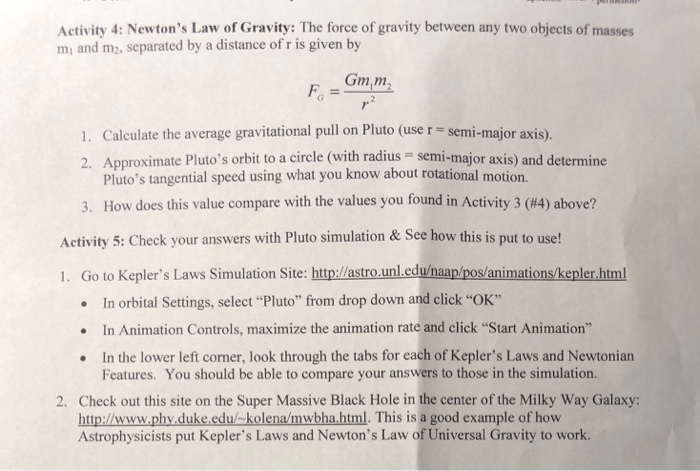I NEED HELP~ ACTIVITY 4 Activity 4: Newton's Law of Gravity: The force of gravity between any two objects of masses mi and m2, separated by a distance of r is given by Gmm 1. Calculate the average gravitational pull on Pluto (use r - semi-major axis). 2. Approximate Pluto's orbit to a circle (with radius semi-major axis) and determine Pluto's tangential speed using what you know about rotational motion. How does this value compare with the values you found...

• ### Multivariable Calculus help with the magnitude of angular momentum: My questions is exercise 4 but I have attached exercise 1 and other notes that I was provided 4 Exercise 4. In any mechanics proble...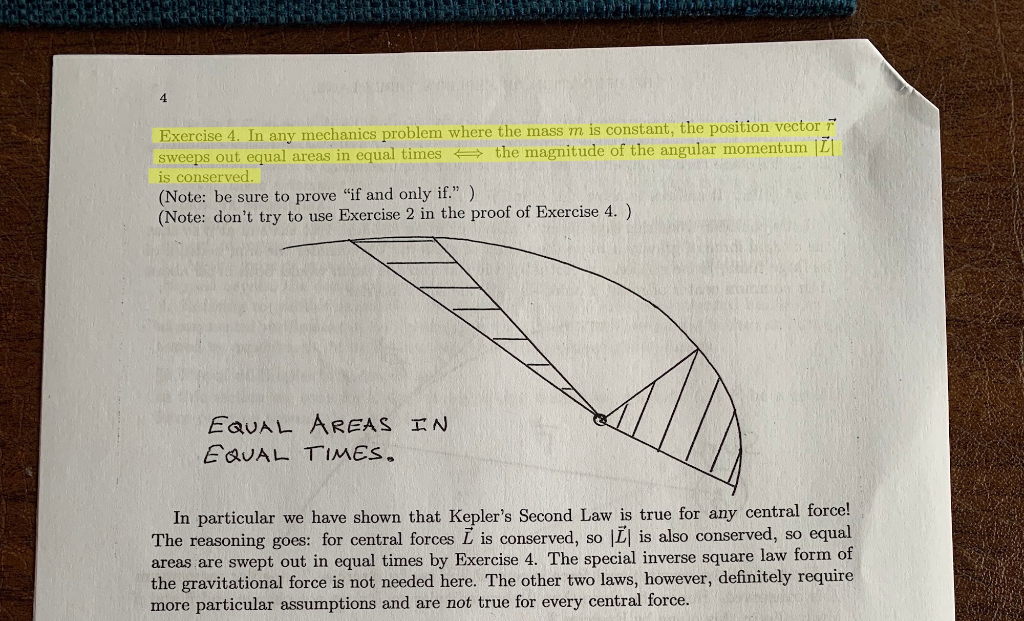Multivariable Calculus help with the magnitude of angular momentum: My questions is exercise 4 but I have attached exercise 1 and other notes that I was provided 4 Exercise 4. In any mechanics problem where the mass m is constant, the position vector F sweeps out equal areas in equal times the magnitude of the angular momentum ILI is conserved (Note: be sure to prove "if and only if") (Note: don't try to use Exercise 2 in the proof of...

• ### 1. Write Newton's 2nd Law in terms of momentum, p. 2. Why is momentum a more...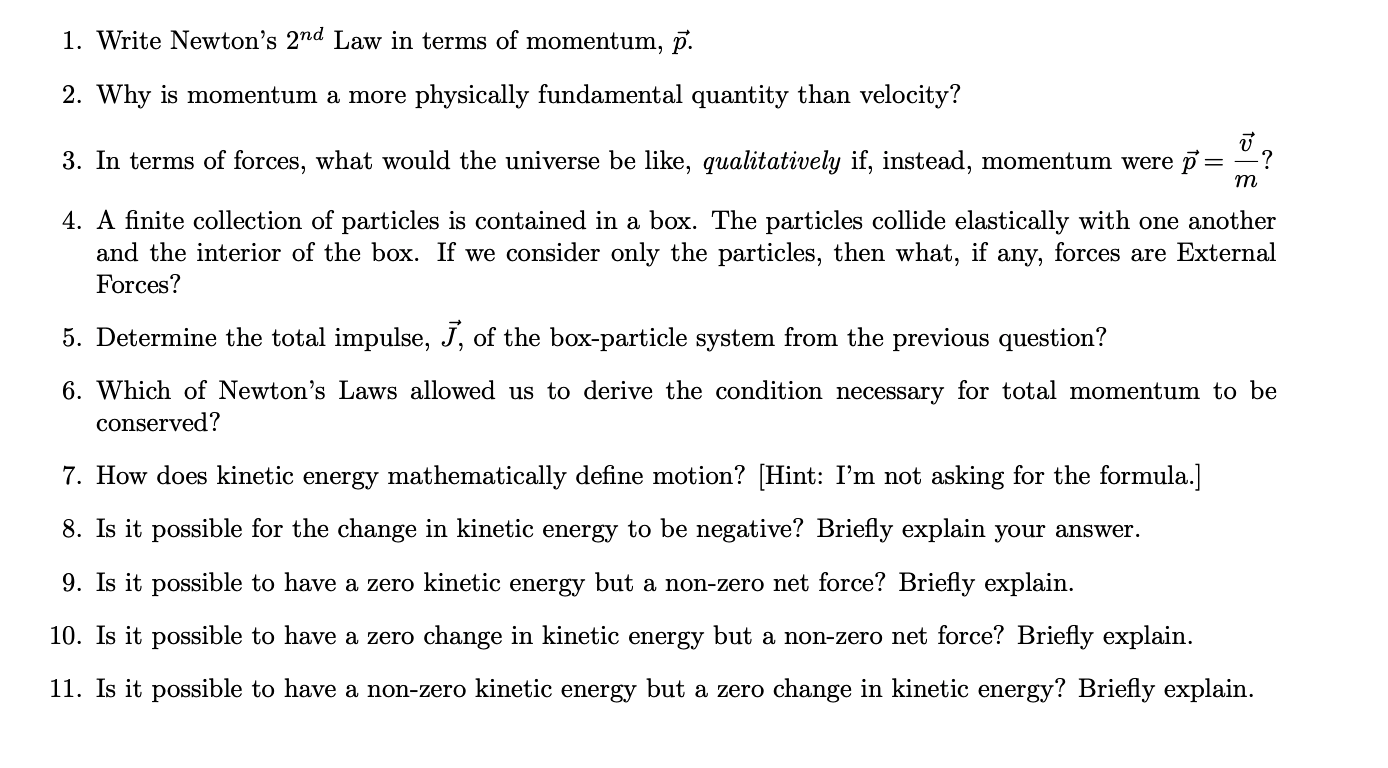1. Write Newton's 2nd Law in terms of momentum, p. 2. Why is momentum a more physically fundamental quantity than velocity? 3. In terms of forces, what would the universe be like, qualitatively if, instead, momentum were p=>? 4. A finite collection of particles is contained in a box. The particles collide elastically with one another and the interior of the box. If we consider only the particles, then what, if any, forces are External Forces? 5. Determine the total...

• ### Q1) Answer all Multiple Choice questions.i) Newton’s 1st law is:A. PHYSICALLY INDEPENDENT of the...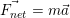Q1) Answer all Multiple Choice questions.i) Newton’s 1st law is:A. PHYSICALLY INDEPENDENT of the other two laws of motion and CANNOT be dispensed with as an axiom of Newtonian physics.B. PHYSICALLY INDEPENDENT of the other two laws of motion, but nonetheless it CAN be dispensed with as an axiom of Newtonian physics.C. Actually a SPECIAL CASE of the 2ND LAW. The case when the net force is zero. Therefore, logically we need only two laws of motion. Perhaps for clarity...

• ### 3. For experiment A, use equations 1&3 to develop a general equation for the value of...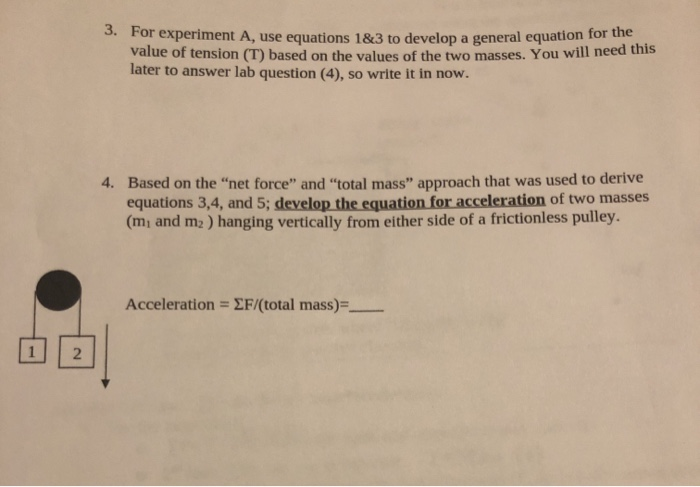3. For experiment A, use equations 1&3 to develop a general equation for the value of tension (T) based on the values of the two masses. You will need this later to answer lab question (4), so write it in now. 4. Based on the "net force" and "total mass" approach that was used to derive equations 3,4, and 5; develop the equation for acceleration of two masses (m, and m2) hanging vertically from either side of a frictionless pulley....